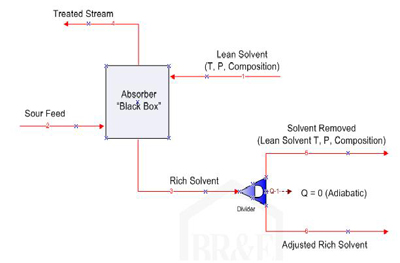# Rich Approach Calculation Method

## Lili Lyddon

### November 30, 2010

Rich Approach is basically the same as an approach to equilibrium, and is intended to give the user a quick idea of whether the circulation rate is suitable. In the 70-80% range, the circulation rate is usually sufficient. If the Rich Approach is above 80% then an upset in the system such as higher acid gas in the feed or increase in feed rate to an amine absorber might lead to a significant increase in acid gas in the treated stream, possibly leading to off-spec gas. Below 70% Rich Approach can indicate over-circulation unless other considerations such as corrosion or foaming require lower loadings.

The Rich Approach calculation is an iterative method which adiabatically removes material from the rich solvent stream as it has been calculated by the staged absorber column until equilibrium is reached. This calculation method can be thought in terms of a “black box” as shown below.Composition and duty of the Lean Solvent stream are removed from the calculated Rich Solvent stream, the enthalpy of the Adjusted Rich Solvent stream is used to calculate the temperature, and finally the temperature and composition are used to calculate the partial pressure of the Adjusted Rich Solvent. This calculation would in effect be similar to using a Divider block which takes a fraction of the specified Lean Solvent stream (say 1% of each and every component in it) and removes that much of each component from the original Rich Solvent flow, and then subtracts the same fraction of the enthalpy of the Lean Solvent from the enthalpy of the Rich Solvent flow (note that when the lean amine is at a higher temperature relative to the rich amine, the calculation sometimes fails for this reason). These iterations continue until the calculated partial pressure of the designated component in the adjusted rich solvent matches the partial pressure of the same component in the sour inlet gas (or liquid) feed stream. At this point equilibrium is assumed.

As an example, if the calculation removed 25% of the Lean Solvent stream from the Rich Solvent stream to achieve equilibrium, then the Rich Approach (or approach to equilibrium loading) would be 75%.

The Rich Approach parameter is only meaningful if the Lean Approach is greater than 90%. In cases where acid gas is slipping (e.g. CO2 in a generic MDEA case, H2S in a case with too few stages to reach equilibrium, etc.), the Rich Approach is not a very useful number as equilibrium would never be achieved, resulting in a conservative or high value.